International
Tables for
Crystallography
Volume D
Physical properties of crystals
Edited by A. Authier

International Tables for Crystallography (2006). Vol. D, ch. 1.1, p. 12

## Section 1.1.4.4.3. Piezoelectricity

A. Authiera*

aInstitut de Minéralogie et de la Physique des Milieux Condensés, Bâtiment 7, 140 rue de Lourmel, 75015 Paris, France
Correspondence e-mail: aauthier@wanadoo.fr

#### 1.1.4.4.3. Piezoelectricity

| top | pdf |

Piezoelectricity, discovered by the Curie brothers (Curie & Curie, 1880), is the property presented by certain materials that exhibit an electric polarization when submitted to an applied mechanical stress such as a uniaxial compression (see, for instance, Cady, 1964; Ikeda, 1990). Conversely, their shape changes when they are submitted to an external electric field; this is the converse piezoelectric effect. The physical interpretation of piezoelectricity is the following: under the action of the applied stress, the centres of gravity of negative and positive charges move to different positions in the unit cell, which produces an electric polarization.

From the viewpoint of symmetry, piezoelectricity can be considered as the superposition of two causes, the crystal with its own symmetry and the applied stress. The symmetry associated with a uniaxial compression is that of two equal and opposite forces, namely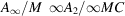. The effect is an electric polarization, of symmetry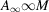, which must be higher than or equal to the intersection of the symmetries of the two causes: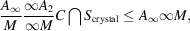where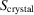denotes the symmetry of the crystal.

It may be noted that the effect does not possess a centre of symmetry. The crystal point groups compatible with the property of piezoelectricity are therefore among the 21 noncentrosymmetric point groups. More elaborate symmetry considerations show further that group 432 is also not compatible with piezoelectricity. This will be proved in Section 1.1.4.10.4using the symmetry properties of tensors. There are therefore 20 point groups compatible with piezoelectricity: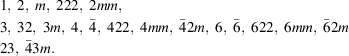The intersection of the symmetries of the crystal and of the applied stress depend of course on the orientation of this stress relative to the crystallographic axes. Let us take, for instance, a crystal of quartz, which belongs to group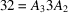. The above condition becomes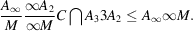If the applied compression is parallel to the threefold axis, the intersection is identical to the symmetry of the crystal,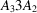, which possesses symmetry elements that do not exist in the effect, and piezoelectricity cannot appear. This is of course obvious because the threefold axis is not polar. For all other directions, piezoelectricity may appear.

### References

Cady, W. G. (1964). Piezoelectricity. New York: Dover.
Curie, J. & Curie, P. (1880). Développement par pression de l'électricité polaire dans les cristaux hémièdres à faces inclinées. C. R. Acad. Sci. 91, 294–295.
Ikeda, T. (1990). Fundamentals of piezoelectricity. Oxford University Press.